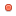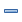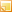###Author Topic: symbol name -> prototype  (Read 897 times)

####OS923##### symbol name -> prototype
« on: March 18, 2019, 04:03:54 AM »
How can I convert a symbol name to a prototype?

Is there a library that can do this?

####OS923##### Re: symbol name -> prototype
« Reply #1 on: March 25, 2019, 04:32:24 AM »
The Fragmalyzer has an SDK with a library. In the header is
Code: [Select]
`pascal void FrazUnmangleSymbol(StringPtr inStMangled, StringPtr outStUnmangled);`That does what I want. But it's a stub library. When the program starts it asks where's the library. Then I changed the type of The Fragmalyzer into 'shlb' and its name into "Fragmalyzer" and this worked, but when the program quits it crashes.

####OS923##### Re: symbol name -> prototype
« Reply #2 on: March 25, 2019, 05:07:53 AM »
I was using The Fragmalyzer 1.1.2 from https://www.info-mac.org/viewtopic.php?f=110&t=2032

I found a more recent version on archive.org (1.5.2). I'm watching this now on a Windows computer. The FragmalyzerLib from 1.5.2 is larger than the libraries from 1.1.2 together. Maybe this will be a shared library.
« Last Edit: March 25, 2019, 05:21:26 AM by OS923 »

####OS923##### Re: symbol name -> prototype
« Reply #3 on: March 26, 2019, 06:04:25 AM »
A possible solution is to write a plugin that adds an Apple event handler to The Fragmalyzer that does the conversion.

####OS923##### Re: symbol name -> prototype
« Reply #4 on: March 27, 2019, 02:50:28 AM »
I found on Wikipedia that it's called "demangle" and that every compiler follows its own scheme. Then I googled epiced "codewarrior demangle symbol" and I found this Python program:

https://gist.github.com/jackoalan/a4035651f6b870136da5

Code: [Select]
`#!/usr/bin/env python# -*- coding: utf-8 -*-import sysdef demangleNode(node):    pointer = False    ref = False    const = False    unsigned = False    while True:        if node == 'P':            pointer = True        elif node == 'R':            ref = True        elif node == 'C':            const = True        elif node == 'U':            unsigned = True        else:            break        node = node[1:]    pre = str()    post = str()    if ref:        post = '&'    if pointer:        post = '*'    if const:        pre = 'const '    if unsigned:        pre += 'unsigned '    if node == 'i':        return pre + 'int' + post, node[1:]    elif node == 'b':        return pre + 'bool' + post, node[1:]    elif node == 'c':        return pre + 'char' + post, node[1:]    elif node == 's':        return pre + 'short' + post, node[1:]    elif node == 'l':        return pre + 'long' + post, node[1:]    elif node == 'f':        return pre + 'float' + post, node[1:]    elif node == 'd':        return pre + 'double' + post, node[1:]    elif node == 'w':        return pre + 'wchar_t' + post, node[1:]    elif node == 'v':        return pre + 'void' + post, node[1:]    elif node == 'F':        return demangleFuncNode(node[1:])    elif node == 'Q':        compCount = int(node)        node = node[2:]        accum = str()        for idx in range(compCount):            demangle, node = demangleNode(node)            accum += demangle + "::"        return pre + accum[:len(accum)-2] + post, node    elif node.isdigit():        counter = 1        while node[counter].isdigit():            counter += 1        length = int(node[:counter])        if node[counter] == '>' or node[counter] == ',':            return '%d' % length, node[counter:]        return pre + node[counter:counter+length] + post, node[counter+length:]    return node, ''def demangleFuncNode(node):    args = str()    while node != '_':        if len(args):            args += ', '        arg, node = demangleNode(node)        args += arg    node = node[1:]    returnType, node = demangleNode(node)    return '%s(%s)' % (returnType, args), nodedef findSepIdx(name, idx):    count = 0    retval = 0    for ch in name:        if ch == '<' or ch == ',':            if idx == count:                return retval            count += 1        retval += 1    return -1def demangleTemplates(name):    token = 0    accum = name    while True:        tidx = findSepIdx(accum, token)        token += 1        if tidx < 0:            return accum        sidx = tidx + 1        toDem = accum[sidx:]        accum = accum[:sidx]        dem, rem = demangleNode(toDem)        accum += dem + remdef demangle(sym):    typeSplit = sym[1:].find('__')    funcName = demangleTemplates(sym[:typeSplit+1])    rem = sym[typeSplit+3:]    funcType, rem = demangleNode(rem)    funcType = demangleTemplates(funcType)    objType = rem    post = str()    if objType == 'C':        post = ' const'        objType = rem        rem = rem[1:]    rem = rem[1:]    funcArgs = str()    while len(rem):        if len(funcArgs):            funcArgs += ', '        arg, rem = demangleNode(rem)        funcArgs += demangleTemplates(arg)    return '%s::%s(%s)%s' % (funcType, funcName, funcArgs, post)if __name__ == "__main__":    if len(sys.argv) < 2:        raise RuntimeError("needs symbol argument")    print(demangle(sys.argv))`

####OS923##### Re: symbol name -> prototype
« Reply #5 on: March 27, 2019, 03:16:02 AM »
The CodeWarrior development kit for PlayStation X had a DEMANGLE.H and SYMMUNGE.OBJ Maybe there's something similar on one of the CodeWarrior install CDs.

####OS923##### Re: symbol name -> prototype
« Reply #6 on: April 09, 2019, 06:17:33 AM »
MPW has an unmangle command.

###10 Post Recent PostsCan anybody help me identify these AVID pci cards? [DAW - Digital Audio Workstation (Audio Interfaces & MIDI Hardware)] devils_advisorToday at 12:57:47 PMMaximum MIDI Ports on MACOS? [DAW - Digital Audio Workstation (Audio Interfaces & MIDI Hardware)] IIOToday at 10:22:30 AMLatest hacks [Hacking the System, Mac OS 9.3, and Beyond !] OS923Today at 09:14:44 AMMac turning on by itself? [General Hardware Discussions] PhilgoodToday at 09:03:58 AMtoast 3 [CD/DVD Authoring & Burning Software] JaklToday at 12:38:58 AMGigaDesigns G4 Upgrade. Audio issues OS 9. [Mac CPU Upgrades] ConorAugust 22, 2019, 04:24:42 PMThe "FrankenMac G4" [General Hardware Discussions] IIOAugust 22, 2019, 03:09:17 PMReCycle 2.1 [Reason / Rebirth / Recycle by Propellerheads] dasmodeAugust 22, 2019, 12:53:15 PMReason Adapted [Reason / Rebirth / Recycle by Propellerheads] dasmodeAugust 22, 2019, 12:49:12 PMSampleCell 2 Plus ISO [Pro Tools by Digidesign] macStuffAugust 22, 2019, 04:58:03 AM